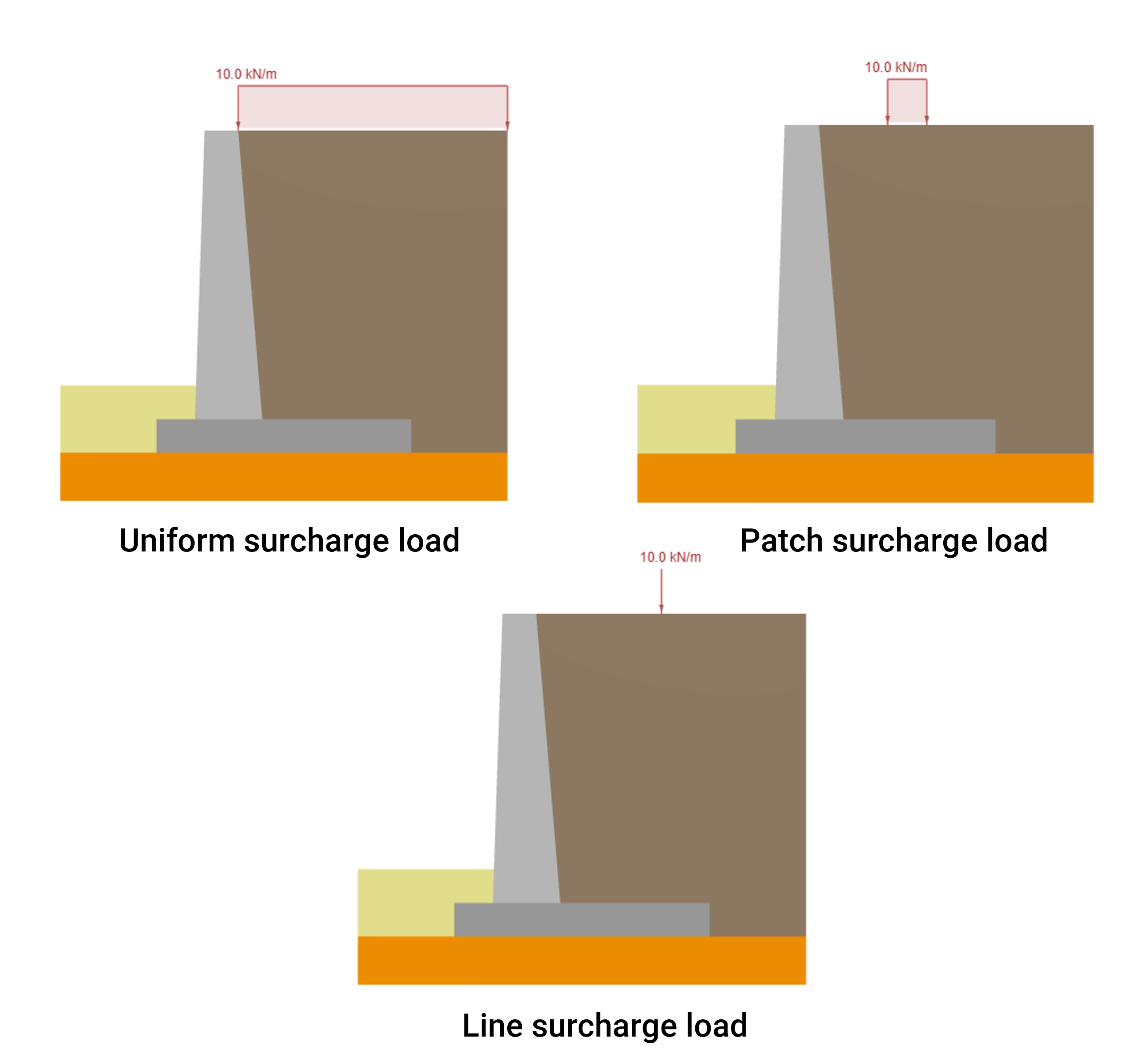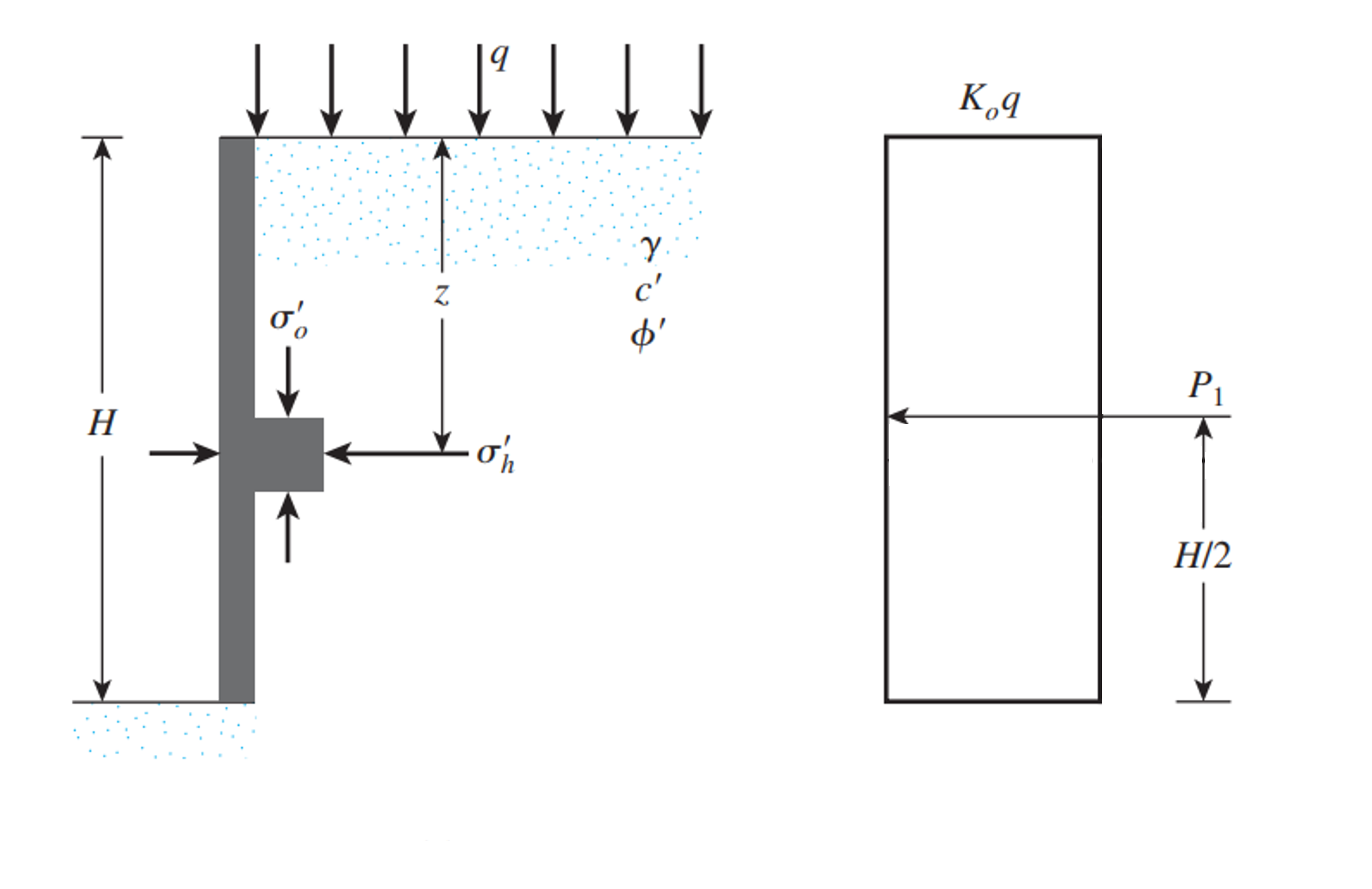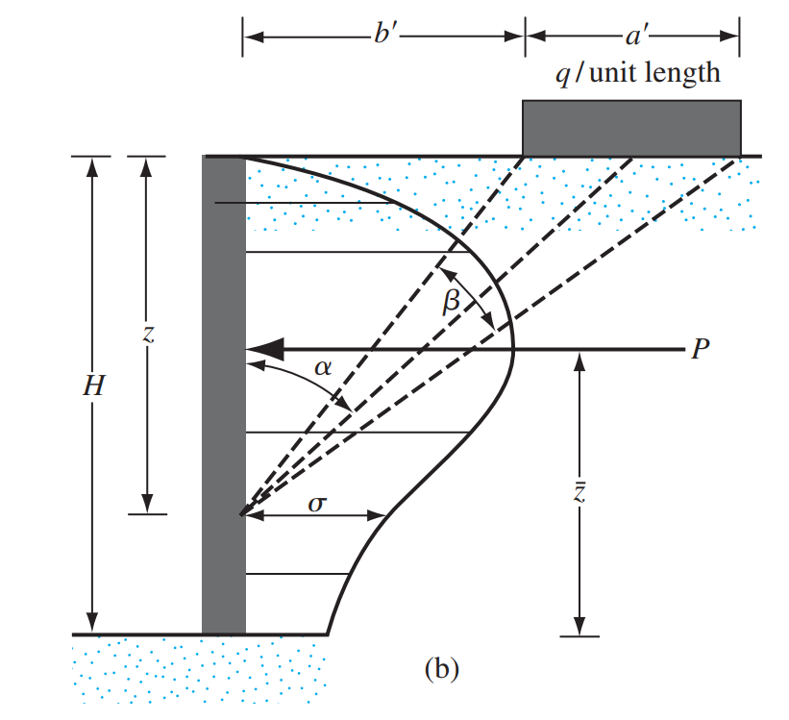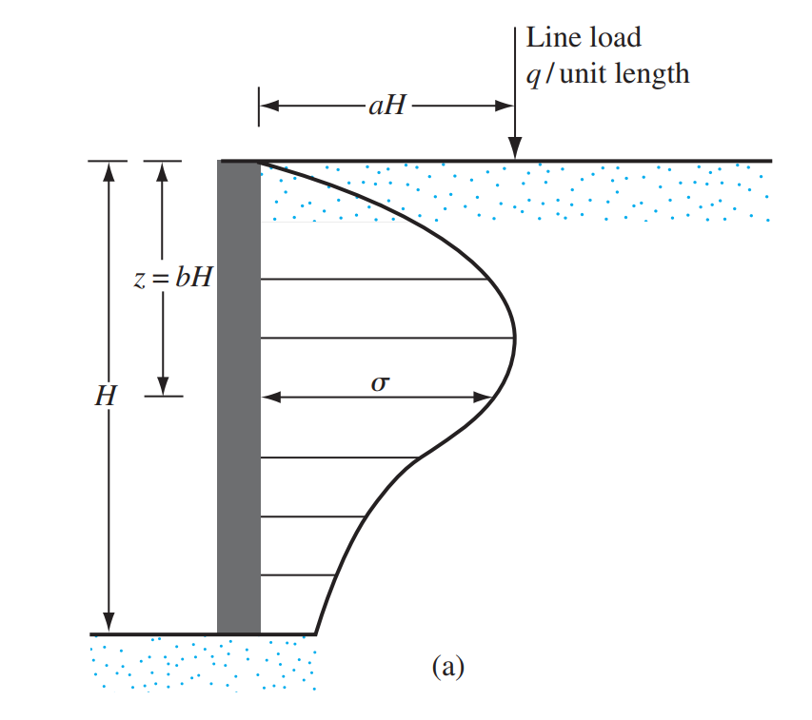SkyCiv文档

1. SkyCiv挡土墙
2. 文章和教程
3. 超载荷载引起的侧向土压力

# 超载荷载引起的侧向土压力

## 计算挡土墙超载荷载引起的侧向土压力

• 计算超载荷载引起的侧向土压力 计算超载荷载引起的侧向土压力
• 计算超载荷载引起的侧向土压力 计算超载荷载引起的侧向土压力
• 计算超载荷载引起的侧向土压力 计算超载荷载引起的侧向土压力### 均匀负载

• 对于静止状态的土壤:

$$P_{区, \; 在休息} = K_{0} \cdot q cdot H_{泥}$$

• 静止 = k_:

$$P_{区, \; 积极的} = K_{一个} \cdot q cdot H_{泥}$$

• 静止 = k_:

$$P_{区, \; 被动的} = K_{p} \cdot q cdot H_{泥}$$### 补丁加载

$$P_{修补} = frac{q}{90} [H(\theta_2- theta_1)]$$

$$\theta_1 = 棕褐色 ^{-1}(\压裂{b'}{H}) (你)$$

$$\theta_2 = 棕褐色 ^{-1}(\压裂{一个’ + b'}{H}) (你)$$

$$\酒吧{与} = H – [\压裂{H^2(\theta_2- theta_1) + (问题) – 57.3啊}{2H(\theta_2- theta_1)}]$$### 线路负载

$$\西格玛 = frac{q}{H} \压裂{0.203 b}{(0.16+ b^2)^ 2}$$ 对于 $$一个 leq 0.4$$$$P_{线} = int_{0}^{H}\西格玛 ,dz = – \压裂{4 高^2个^{2} q}{2 高^2个^{2} \pi + 2 H ^ 2 π} + \压裂{2 q}{\pi} = frac{2 q}{\π左(一个^{2} + 1\对)}$$ 对于 $$一个 > 0.4$$

$$P_{线} = int_{0}^{H}\西格玛 ,dz = 0.546875 q$$ 对于 $$一个 leq 0.4$$

$$\酒吧{与} = H 左(1 – 一个 sqrt{\压裂{1}{2 一个^{2} + 1}}\对)$$ 对于 $$一个 > 0.4$$

$$\酒吧{与} = H (1- 0.348155311911396)$$ 对于 $$一个 leq 0.4$$

## 挡土墙计算器

SkyCiv 提供了一个免费的挡土墙计算器，可以计算墙上的横向土压力, 并对挡土墙进行稳定性分析. 付费版本还显示完整的计算, 所以你可以一步一步看到, 如何计算挡土墙抗倾覆的稳定性, 滑行, 和轴承!ng (民用)